# Inverse laplace transforms

## Inverse Laplace Transform

The inverse Laplace transform definition comes as the inverse operation of the Laplace transformation and is mathematically is written as:

On its integral form, the Inverse Laplace transform looks like:

Although the inverse Laplace transform formula may seem intimidating, we will be showing there are much easier methods to obtain the result for the inverse transform of a function. On this article we will focus on this practical approach of solving the Inverse Laplace transformation, which uses a Laplace transform table as aid. We will call this method "the comparison method" or "table comparison method" and we will show you how to work through it step by step in the next section.

Before you continue, make sure you have had an introduction to the Laplace transform or take a quick review if it has been long since you studied it. It is imperative you have knowledge on calculating Laplace transforms so the terms and methodology on this article are meaningful to your studies.

## How to find the inverse laplace transform:

In order for us to follow our intuitive and practical method of comparison, we have included a table of the most common Laplace transformations.

A nice detail to notice is that you do not need a particular Inverse laplace transform table if you want to solve problems of this type. A general table such as the one below (usually just named a Laplace transform table) will suffice since you have both transforms in there. F(s) is always the result of a Laplace transform and f(t) is always the result of an Inverse Laplace transform, and so, a general table is actually a table of the transform and its inverse in separate columns.

You may be asking yourself, why is it that we need to check Laplace transforms if we are solving Inverse Laplace transforms? Well, as mentioned before, the method we are about to show is intuitive because is all about comparing the table of Laplace transform results to look for equations with similar denominators to the ones we are given during a problem. In that way, we look for an answer on the table to match with the functions we are given to solve, and the solution will be just some kind of form of the function that produced the Laplace transformations in the first place.

Confused? Do not worry, let us look at a few examples so you can see what we are talking about:

Example 1

• Find the inverse Laplace transform of the function by using the table comparison method: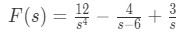Equation for Example 1: Find the Inverse Laplace transform of the given function F(s)
• We divide this function in parts to solve each one separately: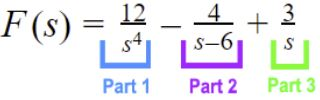Equation for Example 1(a): Dividing the function in parts to solve each part separately
• Part 1:
The first thing we do while solving inverse Laplace transforms by comparison method is to observe the denominators on the given F(s) function. Then we identify a function on the Laplace transform table that has a similar denominator.
Thus, by looking at Part 1 and at table 1, formula C: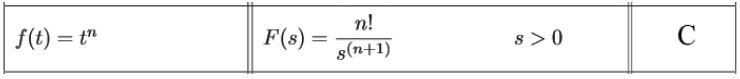Equation for Example 1(b-1): Identifying the general solution of the inverse Laplace transform from the table
• We can easily see how n=3 in this case.
If n=3, then n!=3*2*1=6. Therefore, in order for part 1 to be in the form of formula C we need to factorize some numbers out, and so, part 1 becomes: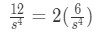Equation for Example 1(b-2): Rearranging terms to solve the equation easier
• Now we take the Inverse Laplace transform: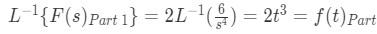Equation for Example 1(b-3): Inverse Laplace transform solution for part 1
• Part 2:
We follow the same steps as before, so we identify a function on the Laplace transform table that has a similar denominator. Thus, by looking at Part 2 and table 1, formula B: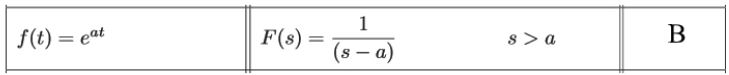Equation for Example 1(c-1): Identifying the general solution of the inverse Laplace transform from the table
• We can easily see how a=6 in this case.
In order for our part 2 to have a denominator equal to 1, we factorize the four out and the equation is: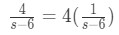Equation for Example 1(c-2): Rearranging terms to solve the equation easier
• Now we take the Inverse Laplace transform: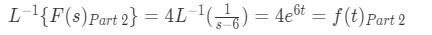Equation for Example 1(c-3): Inverse Laplace transform solution for part 2
• Part 3:
Identify a function on the Laplace transform table that has a similar denominator. Thus, by looking at Part 3 and table 1, formula A: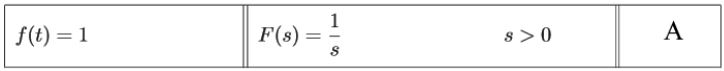Equation for Example 1(d-1): Identifying the general solution of the inverse Laplace transform from the table
• We can easily factorize the 3 out of part 3 so we are left with: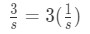Equation for Example 1(d-2): Rearranging terms to solve the equation easier
• To which we take the inverse Laplace transform: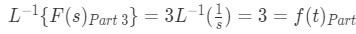Equation for Example 1(d-3): Inverse Laplace transform solution for part 3
• And so we put together all of our answers to obtain the final one:

Example 2

• Find the inverse Laplace transform of the function by using the table comparison method: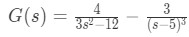Equation for Example 2: Find the Inverse Laplace transform of the given function G(s)
• We divide this function in parts to solve each one separately: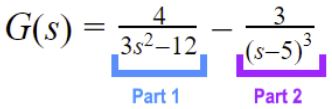Equation for Example 2(a): Dividing the function in parts to solve each part separately
• Part 1:
Identify a function on the Laplace transform table that has a similar denominator. Thus, by looking at Part 1 and table 1, formula F: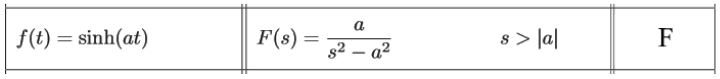Equation for Example 2(b-1): Identifying the general solution of the inverse Laplace transform from the table
• Notice how "a" cannot be 4 or square root of 12. Therefore, in this case we need to find what we can factorize out to simplify part 1 in a way it fits to formula F.
The easy thing to do here is to factorize the 3 out in the denominator: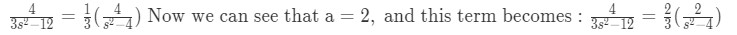Equation for Example 2(b-2): Rearranging terms to solve the equation easier
• Now we take the Inverse Laplace transform: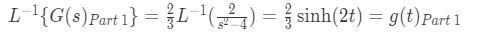Equation for Example 2(b-3): Inverse Laplace transform solution for part 1
• Part 2:
Identify a function on the Laplace transform table that has a similar denominator. Thus, by looking at Part 2 and table 1, formula H: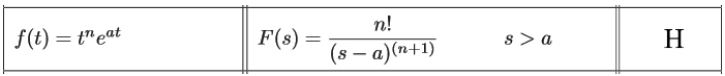Equation for Example 2(c-1): Identifying the general solution of the inverse Laplace transform from the table
• We can rapidly see that n=2 and a=5. Also notice that for n=2, the denominator of part 2 would result as a 2 while we have a 3, and so we need to apply a factor to the term in order to justify this: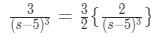Equation for Example 2(c-2): Rearranging terms to solve the equation easier
• Now we take the Inverse Laplace transform: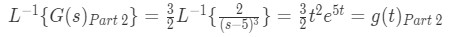Equation for Example 2(c-3): Inverse Laplace transform solution for part 2
• We put together our answers to obtain the Inverse Laplace transform of the whole G(s) function:

After solving two examples, is time for us to list the steps to follow in order to obtain the Inverse Laplace transform of a given function F(s) with the method of table comparison:

1. Check how many terms does the function have, if only one, then the next steps explain how to obtain the inverse Laplace transformation of it. If there are more than one term in the given function, then repeat these steps for each of them.

2. Look at the Laplace transform table and identify a F(s) equation similar (with similar denominator) to the given F(s) in your problem.

3. Identify terms such as "a", "b" and "n" on the given function in relation to the formula on the table, and find their values.

4. Factorize terms out of your given F(s) in a way that the function left is as similar as possible to the F(s) in the table

5. Take the inverse Laplace transform of your simplified function. Find the answer by looking into the function f(t) on the table that gave as a result the F(s) which is similar to the F(s) in your problem.

6. Make sure your answer contains all the proper factors and values for "a", "b" and "n"

So far, we have only used the comparison method to solve the Inverse laplace transform examples, but this is just the tip of the iceberg! For practical purposes we usually have a table (such as table 1) at hand to solve problems quickly. But notice that your table may or may not contain all of the possible Laplace transformations that you need, this can occur due to the type of function that is given to you in a problem. The functions we have solved so far are "nice" and they can all be related easily into the table, but if the denominator happens contain a multiplication of binomials and/or polynomials, your problem may get complicated.

For cases such as the one just described, we may be able to use what we know about partial fractions from algebra. This method will be described in the next section. There may even be other times when you need to explicitly solve the Inverse laplace transform equation, which is usually the case when doing rigorous mathematical calculations and analyses.

## Inverse laplace transform by partial fraction expansion

The partial fraction expansion is actually an add-on to the method of solving the inverse Laplace transformations that we have been seeing. In other words, we will still solve the function given using the table comparison method, but first we need to reduce the expression given to us into a simpler one.

As you have seen in algebra before, the partial fractions technique allows you to decompose a complicated algebraic rational expression into something containing simple terms as denominators only. Once you have simplified the expression into one containing simple denominators, you can go ahead and compare each of these with the table and find your answers for the inverse Laplace transform in the exact same manner as we have done it so far in this article.

Although the method continues to be fairly easy, the computation can get quite lengthy depending on the amount of binomials being multiplied on the denominator of the original expression, since you will have to solve an unknown for each binomial. The more binomials you have the more unknowns you will need to find and the longer the solution to the problem will be.

Let us solve two examples to illustrate not only the methodology for solving the inverse laplace transforms, but also the point we just mentioned in the paragraph above. For these problems you will make use of the partial fractions technique, so we suggest you to study it in advance if you are not familiarized with it, or if you think you need to review it beforehand.

Example 3

• Find the inverse Laplace transform of the next function: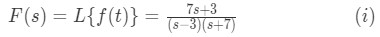Equation for Example 3(a): Find the Inverse Laplace transform of F(s)
• First we solve the partial fractions:
• Multiply the whole expression by the terms found in the denominator on the left hand side to obtain: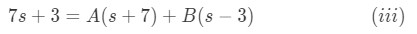Equation for Example 3(c): Simple algebraic equation to solve for values of A and B
• This equation is true for any value of s, thus, in order to find the values of A and B we can set s equal to any number that is convenient to us. Here "convenient" means to set s equal to a value that will make one of the two unknowns vanish, so we can calculate the value of the one that is left. It does not matter which of the two unknowns you select to solve for first.
In this case, we select to set s=-7, therefore the equation (iii) becomes:
• Now inputting this value of B into equation (iii) and setting s=0 we can find A:
• Having the values of A and B, now we can rewrite our function (i) as: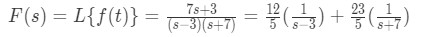Equation for Example 3(g): Rewriting F(s) using the found values of A and B
• And we apply the inverse Laplace transform: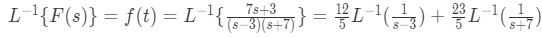Equation for Example 3(h): Inverse Laplace Transform for the simplified F(s)
• Using formula B found in the table: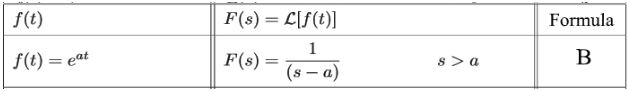Equation for Example 3(i): Identifying the general solution of the inverse Laplace transform from the table
• We can easily see that in this case the solution is: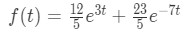Equation for Example 3(j): Finding the final solution of the Inverse Laplace equation using the table-comparison method

Notice we still used the method of comparing with the table of Laplace transformations, that is why this procedure is called inverse Laplace transformations with partial fraction expansion, because we truly just use the same steps as before except for the first part, where we need to expand our techniques in order to simplify the first expression into a polynomial with simple denominators.

Let's continue with the next example in which you will have three binomials on the original denominator.

Example 4

• Obtain the inverse Laplace transform of the next function using the partial fractions expansion.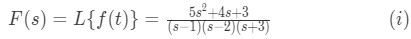Equation for Example 4(a): Find the Inverse Laplace transform of F(s)
• We solve the partial fractions first by establishing 3 unknowns to find (remember the amount of unknowns depends on the amount of binomials in the denominator of equation (i) and if these binomials are simple terms, quadratic, etc). So we rewrite the equation:
• We multiply this whole equation by the terms found in the denominator on the left hand side to obtain: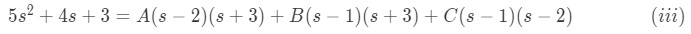Equation for Example 4(c): Simple algebraic equation to solve for values of A, B and C
• And now we set the value of s in a convenient manner so two out of our three unknowns will vanish and we can solve for the one that is left.
Thus, we set s=1, and equation (iii) looks like:
• We substitute the found value of A into equation (iii):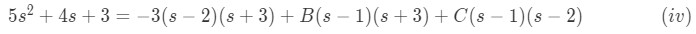Equation for Example 4(e): Inputting the value of A to the equation
• And we set s into another convenient value to solve for one of the two left unknowns.
Set s=2 on equation (iv)
• Substituting the value of B on equation (iv) and now we just set s=0 so we can solve for C:
• We can finally rewrite equation (ii) with the values of A, B and C: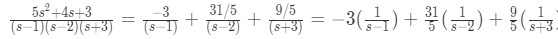Equation for Example 4(h): Rewriting F(s) with the found values of A, B and C
• And take the inverse laplace transform to find f(t):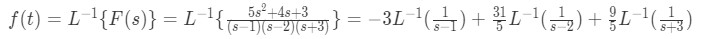Equation for Example 4(i): Inverse Laplace Transform for the simplified F(s)
• We bring back equation B from the Laplace transform table: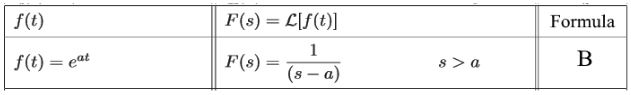Equation for Example 4(j): Identifying the general solution of the inverse Laplace transform from the table
• And by the comparison method we obtain: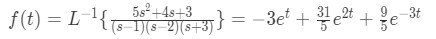Equation for Example 4(k): Finding the final solution of the Inverse Laplace equation using the table-comparison method

If you want to continue your practice we suggest you to visit and read these differential equations notes . Also, the next link contains some more inverse laplace transform examples .

Notice that throughout all of the inverse Laplace transform examples we saw on this article, we did not look at the case in which we obtain the inverse Laplace transform of a constant. This is an special case which will be explained in higher detail on later sections. The reason for this is that the inverse Laplace transform of 1 happens to be the Dirac Delta Function, and thus, trying to solve for the inverse Laplace transform of a constant just gives as a result the constant times the Dirac Delta function.

On such cases we will have to resort to the integral formula for the inverse Laplace transform in order to make the concept of the impulse and diract delta more meaningful. For that reason, we will leave such examples for the article on later sections.

### Inverse laplace transforms

#### Lessons

To solve a differential equation using a Laplace Transform it will also be necessary to know how to use an Inverse Laplace Transform

Finding the Inverse Laplace Transform involves turning the Laplace Transform of a function back into that function.

$L^{-1}{F(s)}$ = $f(t)$

For example from the section Calculating Laplace Transforms we saw,

$L${3$e^{3t}$} = $\frac{3}{s - 3}$

So to find out the inverse of the Laplace Transform of $\frac{3}{s - 3}$:

$L^{-1}${$\frac{3}{s-3}$} = 3$e^{3t}$

The same rule of Linearity applies:

$L^{-1}${$a F(s)$ + $bG(s)$} = $aL^{-1}${$F(s)$} + $bL^{-1}$ {$G(s)$}

• Introduction
What are inverse Laplace Transforms?

• 1.
Inverse Laplace Transforms

Find the inverse Laplace Transform of the following functions:

a)
$F(s)$ = $\frac{12}{s^{4}}$ - $\frac{4}{s - 6}$ + $\frac{3}{s}$

b)
$G(s)$ = $\frac{4}{3s^{2} - 12}$ - $\frac{3}{(s - 5)^{3}}$

• 2.
Calculate the inverse Laplace Transform of the following functions:
a)
$G(s)$ = $\frac{7s - 3}{s^{2} - 2}$

b)
$H(s)$ = $\frac{3s - 5}{s^{2} - 4s + 7}$

• 3.
For each of the following functions calculate their inverse Laplace Transform:
a)
$F(s)$ = $\frac{7s + 3}{(s - 3)(s + 7)}$

b)
$G(s)$ = $\frac{3 s^{2} + 4s - 7}{(s^{2} + 7)(s - 3)}$